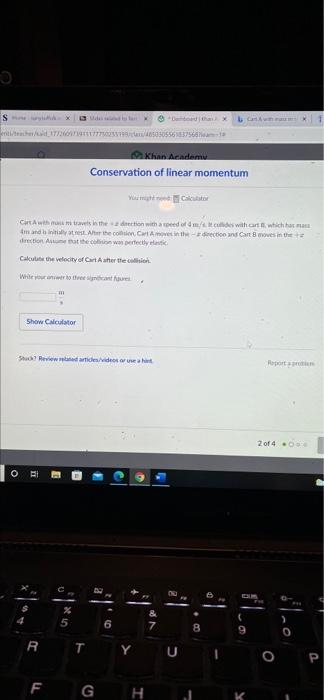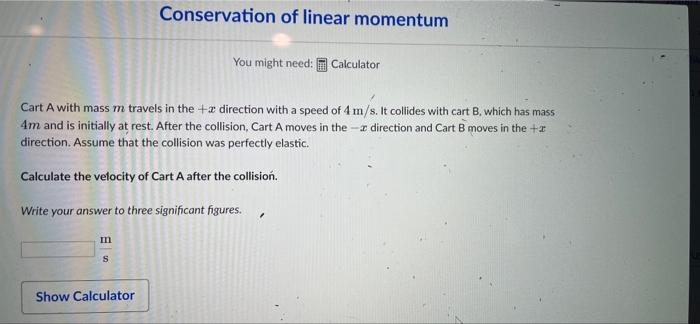# Question Solved1 Answerreposted picture b/c first is blurry S X 030556137 Khan Academy Conservation of linear momentum Cart with me is the direction with a need of me with or which hat man Amandine Alter the content and Cart moves in the direction in the perfectly Calculate the velocity of Cart Attertech m Show Calculator Showbride/videostore 2 of 4 - O 00 6 7 8 lao R T Y U o P F G H Conservation of linear momentum You might need: Calculator Cart A with mass m travels in the + x direction with a speed of 4 m/s. It collides with cart B. which has mass 4m and is initially at rest. After the collision Cart A moves in the -- direction and Cart 8 moves in the + direction. Assume that the collision was perfectly elastic. Calculate the velocity of Cart A after the collision. Write your answer to three significant figures. . m S Show Calculatorreposted picture b/c first is blurryTranscribed Image Text: S X 030556137 Khan Academy Conservation of linear momentum Cart with me is the direction with a need of me with or which hat man Amandine Alter the content and Cart moves in the direction in the perfectly Calculate the velocity of Cart Attertech m Show Calculator Showbride/videostore 2 of 4 - O 00 6 7 8 lao R T Y U o P F G H Conservation of linear momentum You might need: Calculator Cart A with mass m travels in the + x direction with a speed of 4 m/s. It collides with cart B. which has mass 4m and is initially at rest. After the collision Cart A moves in the -- direction and Cart 8 moves in the + direction. Assume that the collision was perfectly elastic. Calculate the velocity of Cart A after the collision. Write your answer to three significant figures. . m S Show Calculator
More
Transcribed Image Text: S X 030556137 Khan Academy Conservation of linear momentum Cart with me is the direction with a need of me with or which hat man Amandine Alter the content and Cart moves in the direction in the perfectly Calculate the velocity of Cart Attertech m Show Calculator Showbride/videostore 2 of 4 - O 00 6 7 8 lao R T Y U o P F G H Conservation of linear momentum You might need: Calculator Cart A with mass m travels in the + x direction with a speed of 4 m/s. It collides with cart B. which has mass 4m and is initially at rest. After the collision Cart A moves in the -- direction and Cart 8 moves in the + direction. Assume that the collision was perfectly elastic. Calculate the velocity of Cart A after the collision. Write your answer to three significant figures. . m S Show Calculator